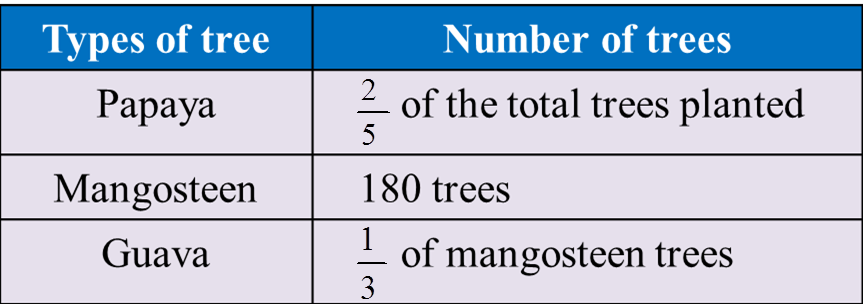3.2.3 Fractions, PT3 Practice

Question 11
:
Table below shows the types of fruit tree planted by Azmi in his orchard.(a) Calculate the number of guava trees.
(b) 100 mangosteen trees died due to fungus.
Find the total number of the remaining trees.

Solution:
(a)

(b)

Question 12:
A palm oil processing factory has a number of workers. $\frac{4}{7}$ of the workers are skilled workers. If $\frac{3}{5}$ of the general workers are 18 females, find the total number of workers in the factory.

Solution:

Question 13:
In a fruit basket, $\frac{2}{5}$ are mangoes and the rest are kiwis.
If $\frac{2}{3}$ of the mangoes or 12 of them are in good condition, calculate the number of kiwis in the basket.

Solution: